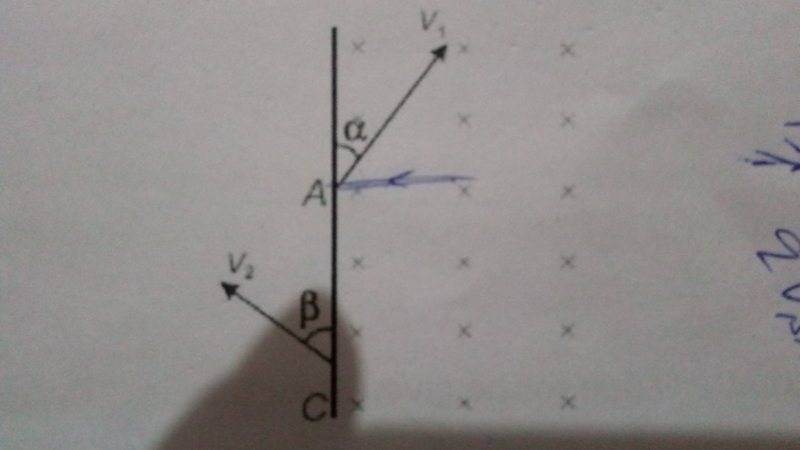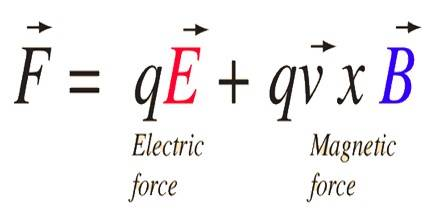# Motion of a charged particle in magnetic field

## Homework Statement

A particle of charge -q and mass m enterd magnetic field B at A with speed v1 at an angle alpha and leaves the field at C with v2 at angle beta.Then,

a)alpha=beta
b)v1=v2
c)particle remains in the field for time t=(2m(pi-alpha))/qB
d)All of these

https://ibb.co/iLMrieF=qvB

## The Attempt at a Solution

Really dont know how to do it.Tried resolving components.

#### Attachments

Last edited by a moderator:

kuruman
Homework Helper
Gold Member
2021 Award

## Homework Statement

A particle of charge -q and mass m enterd magnetic field B at A with speed v1 at an angle alpha and leaves the field at C with v2 at angle beta.Then,

a)alpha=beta
b)v1=v2
c)particle remains in the field for time t=(2m(pi-alpha))/qB
d)All of these

https://ibb.co/iLMrie

F=qvB

## The Attempt at a Solution

Really dont know how to do it.Tried resolving components.[/B]
Resolving components will not get you very far. Other than F=qvB, what else do you know about the motion of particles in magnetic fields? Can you draw the path connecting the entry and exit points of the particle?

berkeman
Mentor
F=qvB
Are you familiar yet with the vector form of that equation (the Lorentz force)? Thinking about the problem in terms of vectors is a lot easier and more intuitive, IMO.#### Attachments

Resolving components will not get you very far. Other than F=qvB, what else do you know about the motion of particles in magnetic fields? Can you draw the path connecting the entry and exit points of the particle?

I dont know to draw the path for particles entering at general angles.just the case when angle=90, 0, and in between.

kuruman
Homework Helper
Gold Member
2021 Award
I dont know to draw the path for particles entering at general angles.just the case when angle=90, 0, and in between.
OK. What kind of path do you get in these cases? Why is the path like that and like something else?

OK. What kind of path do you get in these cases? Why is the path like that and like something else?
Because of magnetic force acting as centripetal force?

kuruman
Homework Helper
Gold Member
2021 Award
Because of magnetic force acting as centripetal force?
Yes., and if the magnetic force is centripetal, what kind of path does the particle describe for as long as the force remains centripetal?

Yes., and if the magnetic force is centripetal, what kind of path does the particle describe for as long as the force remains centripetal?

Depends on the angle velocity makes with the magnetic field.On a general basis i can say circular-kinda motion.

kuruman
Homework Helper
Gold Member
2021 Award
Depends on the angle velocity makes with the magnetic field.On a general basis i can say circular-kinda motion.
Look at your drawing. The magnetic field is directed into the screen and the velocity vector is in the plane of the screen. What do you think the angle between the magnetic field and the velocity is?

Look at your drawing. The magnetic field is directed into the screen and the velocity vector is in the plane of the screen. What do you think the angle between the magnetic field and the velocity is?
The sin alpha component of velocity is only perpendicular
and cos alpha would remain same?

kuruman